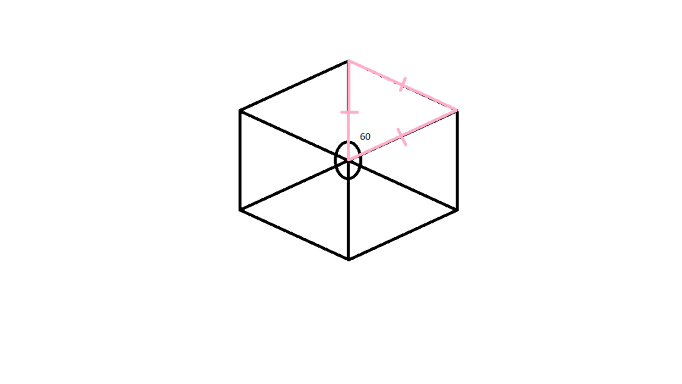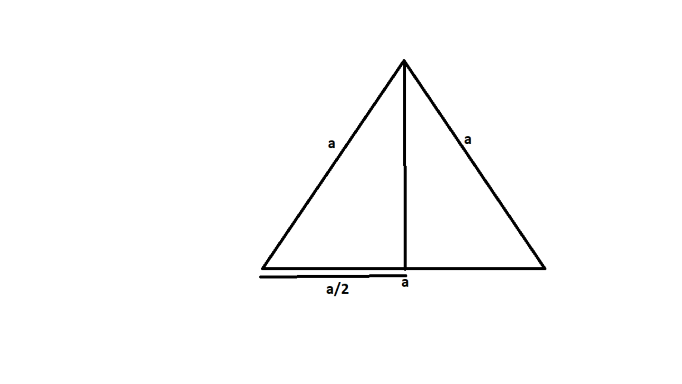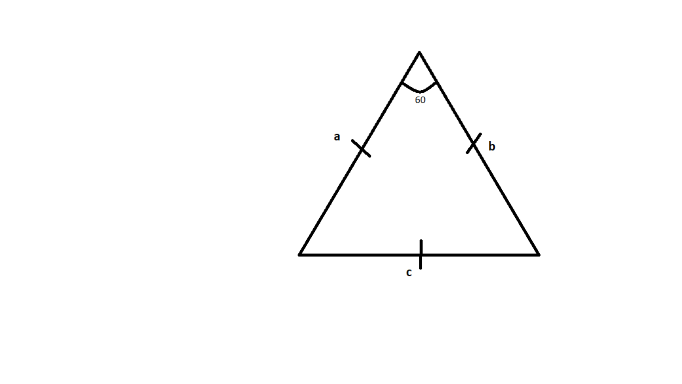# How to Calculate the Area of a Hexagon?

• Last Updated : 29 Jan, 2022

A hexagon is a closed two-dimension geometrical shape. It has six sides of the same or different lengths, six vertices, and six interior angles. In the regular hexagon, all the interior angles are of 120° and exterior angles are of 60°. A regular hexagon has 9 diagonals and can be split into six equilateral triangles. Hexagon is of four types:

• Regular Hexagon: The hexagon having all sides of equal length and with an interior angle 120° is known as a regular hexagon. The diagonals of regular hexagons are equal. They intersect at the center of the hexagon.
• Irregular Hexagon: The hexagon with unequal sides is said to be an irregular hexagon. In an irregular hexagon, all the internal angles are not equal to 120° but their sum is equal to 720°.
• Convex Hexagon: The hexagon having all the vertices pointed outward is known as a convex hexagon. It can be a regular or irregular hexagon and the interior angles are less than 180°.
• Concave Hexagon: The hexagon in which at least one vertex points inward is known as the Concave Hexagon. In this, at least one interior angle is greater than 180°.

### Area of a Hexagon

As we know the hexagon is a geometrical shape and has six sides and vertices. So the area of a hexagon(regular hexagon) is

Area of Hexagon =  3√3/2 x2

Here, x is known as the length of its sides. Here we use a regular hexagon so the length of all the sides is equal.

Derivation for the formula:

As we know a hexagon contains six triangles with a center point as a common vertex. Its area can be found by considering the six times the area of an equilateral triangle.So the area of hexagon = 6 * Area of triangle

As we know the area of triangle  =  1/2 * base * height

where, the base is going to be the one of the side of triangle i.e., a (let)

Now we calculate height using pythagoras theoremFrom the above fig. the vertical line drawn from one of the vertex to the center of base gives the height.

height2 = a2 – (a/2)2 (from pythagoras theorem)

= √3a2/2

Area of triangle  = 1/2 * a * √3a/2

= √3a2/4

Area of hexagon = 6 * Area of  triangle

= 6 * √3/4 * x2

= 3√3/2 * x2

One can consider, the area of triangle =  1/2 *  a *  b * sin ∅ ,

where a is the side of the triangle, b is the side of the triangle and ∅ included angle between the two sides a and bIn equilateral triangle, a = b = x (let) and ∅ = 60°

So the area of equilateral triangle = 1/2 *  x *  x * sin 60°

= 1/2*  x 2 * √3/2 [ sin 60° = √3/2 ]

= √3/4 * x2

Hence the area of hexagon = 6 * Area of Equilateral triangle

= 6 * √3/4 * x2

= 3√3/2 * x2

There isn’t a peculiar method to calculate the area of a regular hexagon. It is calculated by breaking down the regular hexagon into triangles and quadrilaterals and summing up their individual areas at last.

### Area of the hexagon with apothem

We can also calculate the area of a hexagon with apothem. Apothem is a line segment that is drawn from the center and perpendicular to the sides of the hexagon. So the area is

Area of Hexagon = 1/2 x perimeter(hexagon) x apothem

Or we can say

Area of hexagon = 1/2  x 6y x a = 3ya

Here, a is known as apothem and y is known as the length of the sides

### Sample Question

Question 1. Find the area of a hexagon whose side length is 3cm.

Solution:

Given the side of hexagon as 3cm i.e., a = 3cm

Area of hexagon  = 3√3/2 a2

= 3√3/2 (3 * 3)

= 27 √3/2 cm2

Question 2. Find the area of the hexagon with a side length of 2√3 cm.

Solution:

Given the side of hexagon as 3cm i.e., a = 3cm

Area of hexagon  = 3√3/2 a2

= 3√3/2(2√3 * 2√3)

= 18√3 cm2

Question 3. Find the area of a hexagon whose side length is 6cm.

Solution:

Given the side of hexagon as 3cm i.e., a = 6cm

Area of hexagon  = 3√3/2 a2

= 3√3/2(6 * 6)

= 54 √3 cm2

Question 4. Find the area of the hexagon with a side length of 2√6 cm.

Solution:

Given the side of hexagon as 3cm i.e., a = 2√6cm

Area of hexagon  = 3√3/2 a2

= 3√3/2(2√6 * 2√6)

= 36√3 cm2

My Personal Notes arrow_drop_up
Recommended Articles
Page :